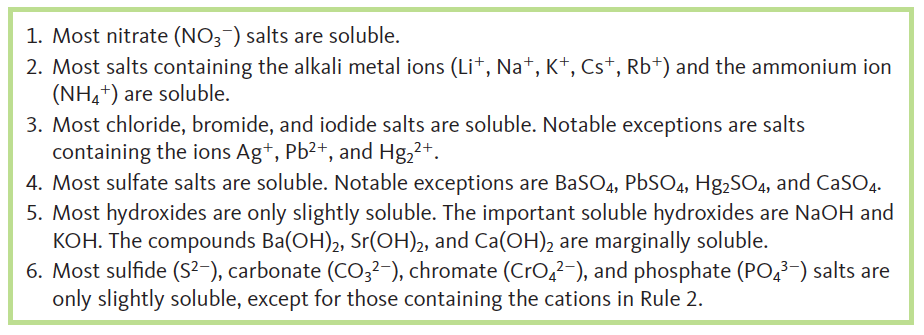# Problem: Using the general solubility rules given below, name three reagents that would form precipitates with calcium ion in an aqueous solution. Write the net ionic equation for each of your suggestions.

###### FREE Expert Solution
96% (160 ratings)
###### Problem Details

Using the general solubility rules given below, name three reagents that would form precipitates with calcium ion in an aqueous solution. Write the net ionic equation for each of your suggestions.